# SVG In, SVG Out

Version 2: Tuesday 14 April 2020

Version 1: original publicationThis report discusses how to work with complex SVG images in R. We look at importing an external SVG image into R with the 'grImport2' package, integrating the imported image with other R graphics, such as plots, and exporting the result to an external SVG image with the 'gridSVG' package. We discuss some of the complications that can arise with this workflow and show that Version 0.2-0 of the 'grImport2' package helps to deal with those complications.

## 1. Introduction

The 'grImport2' package (Potter and Murrell, 2019) can be used to import SVG images into R. For example, the following code imports the SVG logo and draws it in R graphics. The steps involved are: convert the SVG image to a Cairo-based SVG image (one way to do this is with the 'rsvg' package; Ooms, 2018); read the Cairo-based SVG into R with `readPicture()`; and draw the image in R with `grid.picture()`.

```library(grImport2)
library(rsvg)
rsvg_svg("svg-logo-v.svg", "svg-logo-v-cairo.svg")
grid.picture(SVGlogo)
```The value of being able to import external SVG images like this is that you can then integrate them with other R graphics, such as plots. For example, the following code places the SVG logo in the top-right corner of a 'lattice' plot (Sarkar, 2008). This technique can be used, for example, to add a company or institution logo to a plot.

```library(lattice)
xyplot(mpg ~ disp, mtcars,
panel=function(...) {
panel.xyplot(...)
grid.picture(SVGlogo, x=1, y=1, just=c("right", "top"),
width=unit(2, "cm"), height=unit(2, "cm"))
})
```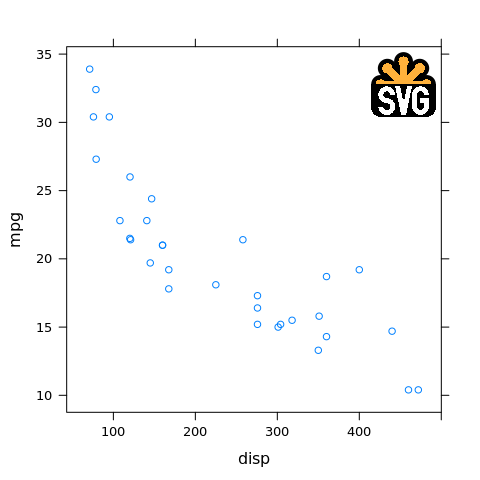Producing SVG output from R is useful because SVG is a vector format, so it produces a nicer (smoother) image at any size. The plot above is a PNG image and we can see, for example, jagged edges on the letters in the SVG logo. The plot below is an SVG version, produced using the `svg()` device, and the result is much smoother.In summary, it is useful to be able to import SVG images into R because we may want to include images that have been created outside of R as part of an R plot. It is also useful to be able to generate SVG output from R because that produces the best visual result in web pages.

## 2. Complex SVG

External SVG images may contain more sophisticated graphical features. For example, the R logo, shown below, consists of two paths, each of which is filled with a (subtle) colour gradient.The 'grImport2' package will happily import images with more sophisticated features, but drawing the image in R is a problem because R graphics does not support some of these features.

For example, the following code imports the R logo (SVG format) and draws it within R, but because R graphics does not support colour gradient fills, drawing the R logo produces no output at all!

```rsvg_svg("Rlogo.svg", "Rlogo-cairo.svg")
grid.picture(Rlogo)
grid.rect()
```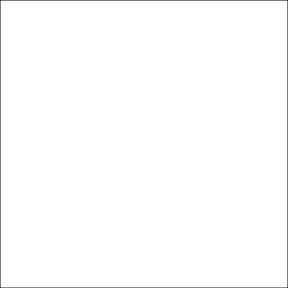The following code demonstrates that we have imported the R logo correctly. In this code, we override the colour gradient fills in the original image and just draw the outlines of the two paths that we have imported from the logo.

```grid.picture(Rlogo, gpFUN=function(gp) { gpar(col="black") })
```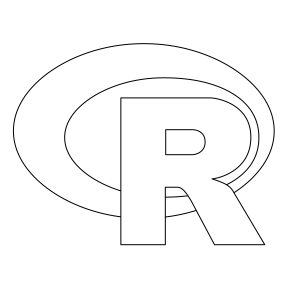The 'gridSVG' package (Murrell and Potter, 2019) can help us here. This package can export ('grid') R graphics in SVG format including sophisticated graphics features. The following code draws the R logo again, in SVG format, but this time it uses 'gridSVG' to do the drawing. The steps involved are: open a 'gridSVG' graphics device, with `gridsvg()`; and supply `ext="gridSVG"` to `grid.picture()`.

```library(gridSVG)
gridsvg("Rlogo-gridSVG.svg", width=3, height=3, res=96)
grid.picture(Rlogo, ext="gridSVG")
dev.off()
```The following code demonstrates the full value of these tools (at least conceptually): an external SVG image, including sophisticated features, has been imported into R, integrated with an R plot, and exported in SVG format, complete with sophisticated features.

```gridsvg("Rplot-gridSVG.svg", width=5, height=5, res=96)
xyplot(mpg ~ disp, mtcars,
panel=function(...) {
panel.xyplot(...)
grid.picture(Rlogo, x=1, y=1, just=c("right", "top"),
width=unit(2, "cm"), height=unit(1.55, "cm"),
ext="gridSVG")
})
dev.off()
```## 3. Context-sensitive SVG

This section side-tracks into a discussion of some of the details about sophisticated SVG graphics features. We might not choose to make use of these details deliberately when generating our own SVG images, but they become important when we import an external SVG image because we have no control over whether the person who created the external image has made use of these details.

A complication that can arise when generating SVG from R is the export of "context-sensitive" graphics features, such as an SVG mask. A mask is a shape that is used to affect the transparency of another shape; wherever the mask is white, the other shape is opaque, wherever the mask is black, the other shape is transparent and (what makes masks different from clipping paths), wherever the mask is grey, the other shape is translucent.

The following code and images demonstrate how a mask works. We will work with a mask that consists of three vertical bars side by side, with one filled black, one filled grey, and one filled white.

```maskShape <- rectGrob(x=0:2/3, width=1/3, just="left",
gp=gpar(col=NA, fill=c("black", "grey", "white")))
```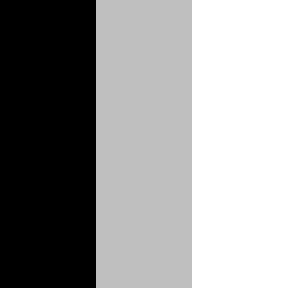The shape that we are going to apply this mask to is a red circle, with the name `"c"`.

```shape <- circleGrob(name="c", r=.4, gp=gpar(col=NA, fill=hcl(0, 60, 60)))
```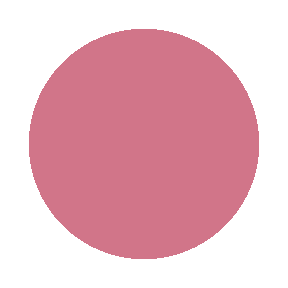The following code generates an SVG image consisting of a blue background, with the red circle drawn on top, and the mask applied to the red circle. The steps involved are: "register" the mask and associate it with a label; draw the shape that we want to mask; and apply the mask to the shape (referring to the shape by its name and the mask by its label). The result is that the left slice of the circle becomes fully transparent, the middle slice of the circle becomes translucent (the result is a mix of the red circle and the blue background), and the right slice of the circle is fully opaque.

```gridsvg("mask.svg", width=3, height=3, res=96)
grid.rect(gp=gpar(col=NA, fill=hcl(240, 60, 60)))
grid.draw(shape)
dev.off()
```The next examples demonstrate the idea of "context sensitivity". First of all, we will modify the code to draw the circle (and apply the mask) within a 'grid' viewport that only occupies the right half of the image. The result is that most of the circle is opaque because the mask that we are applying was registered relative to the whole image - the mask occupies all of the image while the circle only occupies the right half of the image.

```gridsvg("mask-page.svg", width=3, height=3, res=96)
grid.rect(gp=gpar(col=NA, fill=hcl(240, 60, 60)))
pushViewport(viewport(x=.5, width=.5, just="left"))
grid.draw(shape)
dev.off()
```

In the following code, we register the mask within the viewport as well as drawing the circle within the viewport. The result now is a smaller version of the original masked circle because the mask that we are applying was registered relative to the viewport - both the mask and the circle only occupy the right half of the image.

```gridsvg("mask-vp.svg", width=3, height=3, res=96)
grid.rect(gp=gpar(col=NA, fill=hcl(240, 60, 60)))
pushViewport(viewport(x=.5, width=.5, just="left"))
grid.draw(shape)
dev.off()
```

While this level of control is interesting and powerful, it may not be something we choose to make use of deliberately. However, when we import an external SVG image with 'grImport2' whether we end up with an image that makes use of these "context-sensitive" features, like masks, is out of our control.

## 4. Exporting imported context-sensitive SVG

In this section, we bring together the import of SVG images with sophisticated and context-sensitive graphics features and the export of those SVG images (to SVG).

The following image is a diagram that was drawn in Adobe Illustrator (thanks to Artem Sokolov). The original image was PDF, but we cannot directly import PDF images, so the image has been converted to a Cairo-based SVG version using the `pdf2svg` tool (Barton and Flaschen, 2013). The goal is to import this image into R and combine it with an R plot.An important feature of this SVG image is that that light blue fill is achieved by way of an SVG mask (and an SVG filter) applied to an opaque dark blue fill. This is an example of an image that contains sophisticated context-sensitive features over which we have no control. The presence of the mask (and filter) becomes apparent when we import the image to R and attempt to render it with R graphics. R graphics supports translucent colours, but it does not support masks (or filters), so we only get the dark blue fill from the SVG image when we draw it in R.

```test1 <- readPicture("test1.svg")
grid.picture(test1)
```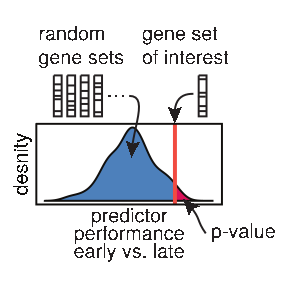The following code demonstrates that we can fix the problem by exporting the imported image to SVG using 'gridSVG', which does support masks (and filters). The `delayContent` argument is significant, but it will be explained later.

```gridsvg("test1-gridSVG.svg", width=3, height=3, res=96)
grid.picture(test1, ext="gridSVG", delayContent=FALSE)
dev.off()
```The following code draws the imported image again (in SVG format, using 'gridSVG'), but this time the imported image is combined with a 'ggplot2' plot (Wickham, 2016) using the 'cowplot' package (Wilke, 2018). We have to call `pictureGrob()` rather than `grid.picture()` because we need a 'grid' grob to pass to `plot_grid()`. The result is not good - part of the imported image has disappeared!

```library(cowplot)
```
```gridsvg("test1-gridSVG-right.svg", width=6, height=3, res=96)
grid.rect(gp=gpar(col=NA, fill="grey80"))
ggplot <- qplot(disp, mpg, data=mtcars)
test1grob <- pictureGrob(test1, ext="gridSVG", delayContent=FALSE)
plot_grid(ggplot, test1grob)
dev.off()
```This is a manifestation of context-sensitive SVG features (when exporting to SVG with 'gridSVG'); it is just a more complicated example of the circle drawn on half the page masked by a mask that is relative to the whole page from the previous section. The problem is that the mask (and filter) within the imported image is being registered when we call `pictureGrob()`, which is relative to the whole page, but the imported image is being drawn by 'cowplot' in only half of the page.

The solution is to make sure that the masks (and filters) in the imported image are registered in the correct viewport; just like when the circle and the mask were both relative to half the page in the previous section. The way that we do that is by specifying `delayContent=TRUE` to `pictureGrob()`; this means that registration only happens when the imported image is drawn (not when we call `pictureGrob()`), which means that registration happens in the correct viewport. The code below demonstrates this and the resulting image is now correct.

```gridsvg("test1-gridSVG-right-delay.svg", width=6, height=3, res=96)
grid.rect(gp=gpar(col=NA, fill="grey80"))
ggplot <- qplot(disp, mpg, data=mtcars)
test1grob <- pictureGrob(test1, ext="gridSVG", delayContent=TRUE)
plot_grid(ggplot, test1grob)
dev.off()
```In the latest version of 'grImport2' (version 0.2-0), `delayContent=TRUE` is the default when `ext="gridSVG"`, so the right thing should happen automatically, without us having to specify the `delayContent` argument explicitly. The following code will also produce the correct result.

```gridsvg("test1-gridSVG-right-delay.svg", width=6, height=3, res=96)
grid.rect(gp=gpar(col=NA, fill="grey80"))
ggplot <- qplot(disp, mpg, data=mtcars)
test1grob <- pictureGrob(test1, ext="gridSVG")
plot_grid(ggplot, test1grob)
dev.off()
```

## 5. Summary

SVG images can contain sophisticated graphics features. The 'grImport2' package allows us to import SVG images that contain sophisticated features into R. The 'gridSVG' package allows us to export SVG images that contain sophisticated features. Version 0.2-0 of the 'grImport2' package makes sure that when we import an SVG image, combine the imported image with other R graphics, and then export an SVG image, the imported image is exported correctly.

## 6. Technical requirements

The examples and discussion in this document relate to grImport2_0.2-0, which is available from R-Forge, and gridSVG_1.7-1, which is available from CRAN.

This report was generated within a Docker container (see Resources section below).

## 7. Resources

• The raw source file for this report, a valid XML transformation of the source file, a 'knitr' document generated from the XML file, two R files and the bibtex file that are used to generate the table of contents and reference sections, two XSL files and an R file that are used to transform the XML to the 'knitr' document, and a Makefile that contains code for the other transformations and coordinates everything. These materials are also available on github.
• This report was generated within a Docker container. The Docker command to build the report is included in the Makefile above. The Docker image for the container is available from Docker Hub; alternatively, the image can be rebuilt from its Dockerfile.

## How to cite this document

Murrell, P. (2019). "SVG In, SVG Out" Technical Report 2019-02, Department of Statistics, The University of Auckland. Version 2. [ bib | DOI | http ]

## 8. References

[Barton and Flaschen, 2013]
Barton, D. and Flaschen, M. (2013). pdf2svg. [ bib | http ]
[Murrell and Potter, 2019]
Murrell, P. and Potter, S. (2019). gridSVG: Export 'grid' Graphics as SVG. R package version 1.7-1. [ bib ]
[Ooms, 2018]
Ooms, J. (2018). rsvg: Render SVG Images into PDF, PNG, PostScript, or Bitmap Arrays. R package version 1.3. [ bib | http ]
[Potter and Murrell, 2019]
Potter, S. and Murrell, P. (2019). grImport2: Importing 'SVG' Graphics. R package version 0.2-0. [ bib ]
[R Core Team, 2019]
R Core Team (2019). R: A Language and Environment for Statistical Computing. R Foundation for Statistical Computing, Vienna, Austria. [ bib | http ]
[Sarkar, 2008]
Sarkar, D. (2008). Lattice: Multivariate Data Visualization with R. Springer, New York. ISBN 978-0-387-75968-5. [ bib | http ]
[Wickham, 2016]
Wickham, H. (2016). ggplot2: Elegant Graphics for Data Analysis. Springer-Verlag New York. [ bib | http ]
[Wilke, 2018]
Wilke, C. O. (2018). cowplot: Streamlined Plot Theme and Plot Annotations for 'ggplot2'. R package version 0.9.3. [ bib | http ]